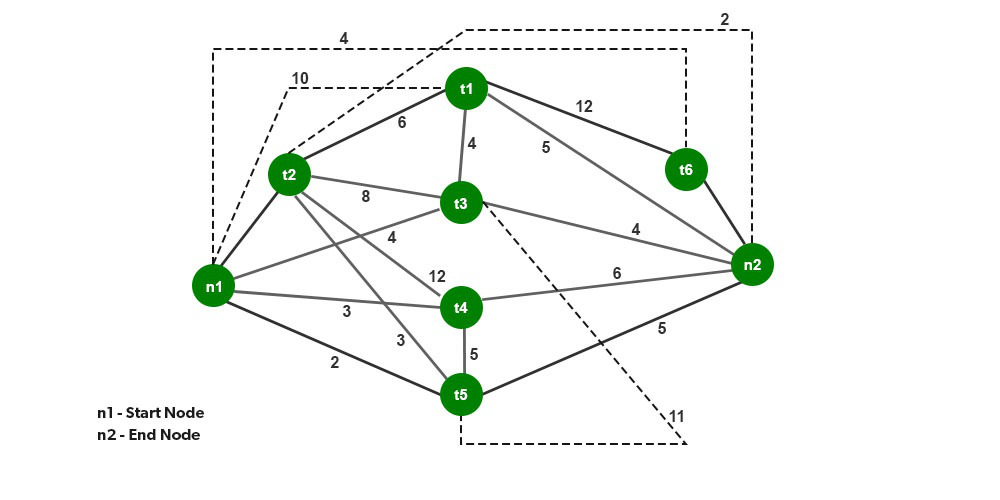GFG App
Open AppBrowser
Continue

# What is Task Assignment Approach in Distributed System?

A Distributed System is a Network of Machines that can exchange information with each other through Message-passing. It can be very useful as it helps in resource sharing. In this article, we will see the concept of the Task Assignment Approach in Distributed systems.

### Resource Management:

One of the functions of system management in distributed systems is Resource Management. When a user requests the execution of the process, the resource manager performs the allocation of resources to the process submitted by the user for execution. In addition, the resource manager routes process to appropriate nodes (processors) based on assignments.

Multiple resources are available in the distributed system so there is a need for system transparency for the user. There can be a logical or a physical resource in the system. For example, data files in sharing mode, Central Processing Unit (CPU), etc.

As the name implies, the task assignment approach is based on the division of the process into multiple tasks. These tasks are assigned to appropriate processors to improve performance and efficiency. This approach has a major setback in that it needs prior knowledge about the features of all the participating processes. Furthermore, it does not take into account the dynamically changing state of the system. This approach’s major objective is to allocate tasks of a single process in the best possible manner as it is based on the division of tasks in a system. For that, there is a need to identify the optimal policy for its implementation.

### Working of Task Assignment Approach:

In the working of the Task Assignment Approach, the following are the assumptions:

• The division of an individual process into tasks.
• Each task’s computing requirements and the performance in terms of the speed of each processor are known.
• The cost incurred in the processing of each task performed on every node of the system is known.
• The IPC (Inter-Process Communication) cost is known for every pair of tasks performed between nodes.
• Other limitations are also familiar, such as job resource requirements and available resources at each node, task priority connections, and so on.

### Goals of Task Assignment Algorithms:

• Reducing Inter-Process Communication (IPC) Cost
• Quick Turnaround Time or Response Time for the whole process
• A high degree of Parallelism
• Utilization of System Resources in an effective manner

The above-mentioned goals time and again conflict. To exemplify, let us consider the goal-1 using which all the tasks of a process need to be allocated to a single node for reducing the Inter-Process Communication (IPC) Cost. If we consider goal-4 which is based on the efficient utilization of system resources that implies all the tasks of a process to be divided and processed by appropriate nodes in a system.

Note: The possible number of assignments of tasks to nodes:

`For m tasks and n nodes= m x n`

But in practice, the possible number of assignments of tasks to nodes < m x n because of the constraint for allocation of tasks to the appropriate nodes in a system due to their particular requirements like memory space, etc.

### Need for Task Assignment in a Distributed System:

The need for task management in distributed systems was raised for achieving the set performance goals. For that optimal assignments should be carried out concerning cost and time functions such as task assignment to minimize the total execution and communication costs, completion task time, total cost of 3 (execution, communication, and interference), total execution and communication costs with the limit imposed on the number of tasks assigned to each processor, and a weighted product of cost functions of total execution and communication costs and completion task time. All these factors are countable in task allocation and turn, resulting in the best outcome of the system.

### Example of Task Assignment Approach:

Let us suppose, there are two nodes namely n1 and n2, and six tasks namely t1, t2, t3, t4, t5, and t6. The two task assignment parameters are:

• execution cost: xab refers to the cost of executing a task an on node b.

Note: The execution of the task (t2) on the node (n2) and the execution of the task (t6) on the node (n1) is not possible as it can be seen from the above table of Execution costs that resources are not available.

### Case1: Serial Assignment

Cost of Execution in Serial Assignment:

``` t11 + t21 + t31 + t42 + t52 + t62  = 5 + 2+ 4 + 3 + 2 + 4
= 20 (Refer Execution Cost table)```

Cost of Communication in Serial Assignment:

```= c14 + c15 + c16 + c24 + c25 + c26 + c34 + c35 + c36
= 0 + 0+ 12 + 12 + 3 + 0 + 0 + 11 + 0
= 38 (Refer Inter-task Communication Cost table)

Hence, Total Cost in Serial Assignment
= 20 + 38
= 58                                                                           ```

### Case2: Optimal Assignment

Cost of Execution in Optimal Assignment:

```= t11 + t21 + t31 + t41 + t51 + t62
= 5 + 2+ 4 + 6 + 5 + 4
= 26 (Refer Execution Cost table)```

Cost of Communication in Optimal Assignment:

```= c16 + c26 + c36 + c46 + c56
= 12 + 0+ 0 + 0 + 0
= 12 (Refer Inter-task Communication Cost table)

Hence, Total Cost in Optimal Assignment
= 26 + 12
= 38   ```

### Optimal Assignment using Minimal Cutset:

Cutset: The cutset of a graph refers to the set of edges that when removed makes the graph disconnected.

Minimal Cutset: The minimal cutset of a graph refers to the cut which is minimum among all the cuts of the graph.My Personal Notes arrow_drop_up